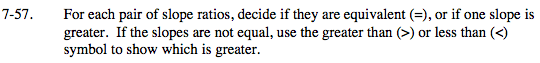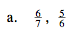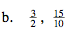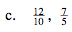### Home > MC2 > Chapter Ch7 > Lesson 7.1.6 > Problem7-57

7-57.Find a common denominator
for the fractions.
Let's use 42.

$\text{Multiply \frac{6}{7}\: by\: \frac{6}{6}\: and\: \frac{5}{6}\: by\: \frac{7}{7}.}$

Compare to find the greatest fraction.